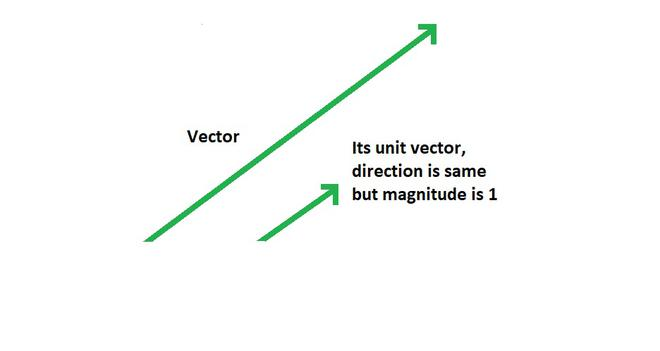# How to calculate the Unit Vector?

Physical quantities are divided into two types: “vector” and “scalar”.The term “vector” refers to a physical quantity that has magnitude as well as direction. These are the physical quantities that follow the triangle law of vector addition. Some of the examples of vector quantities are Electric field, displacement, momentum, velocity, force, and acceleration. All of these quantities have both magnitudes as well as direction. “Scalar” quantities, on the other hand, have only magnitude. Some of the examples of scalar quantities are distance, length, volume, temperature, and area.

### Types of vector

• Equal vectors: Vectors having the same magnitude and same direction are called equal vectors.
• Collinear vectors: Vectors that are either in the same direction or in the opposite direction of each other are called collinear vectors.
• Parallel vectors: Parallel vectors are also known as like vectors. Collinear vectors with similar directions are called parallel vectors. The angle between these vectors is zero.
• Antiparallel vectors: Antiparallel vectors are also known as, unlike vectors. Collinear vectors with opposite directions are called antiparallel vectors. The angle between these vectors is 180°.
• Coplanar vectors: All vectors that lie in the same plane are known as Coplanar vectors.
• Zero vectors: A vector with the same initial point and the terminal point is known as a zero vector. It is also called Null vector. The magnitude of such a vector is 0 and its direction is indeterminant.

### Calculating Unit Vector

A unit vector is a vector of magnitude 1 and with a direction along a given vector. It represents the direction of the given vector. The unit vector of a vector is found by dividing the vector by its modulus. The modulus of a vector is the magnitude of the vector.It is represented by symbol ‘‘(hat or cap) over a variable asand is given by,Where |A| is the modulus of vector A and for a vector, |A| is given by,Thus for a vector, unit vector is given by,### Sample Problems

Problem 1: Given. Find.

Solution:

Modulus of the vector,= √9

= 3

Unit vector,Problem 2: Is a vector given byalso a unit vector?

Solution:

Modulus of the vector,= √3

Magnitude of this vector is not 1. Hence, it is not a unit vector.

Problem 3: Find the unit vector in the direction of.

Solution:

Modulus of the vector,= √3

Unit vector,Problem 4: Ifis a unit vector then find the value of z.

Solution:

Magnitude of a unit vector is 1, which means:which means,Squaring both sides,Question 5: Find unit vector of.

Solution:

Modulus of the vector,Unit vector,Question 6: Find unit vector along.

Solution:

Modulus of the vector,Unit vector,Question 7: If unit vector alongof magnitude 2√2 is. Find.

Solution:

Unit vector,Which meansThus,Whether you're preparing for your first job interview or aiming to upskill in this ever-evolving tech landscape, GeeksforGeeks Courses are your key to success. We provide top-quality content at affordable prices, all geared towards accelerating your growth in a time-bound manner. Join the millions we've already empowered, and we're here to do the same for you. Don't miss out - check it out now!

Previous
Next